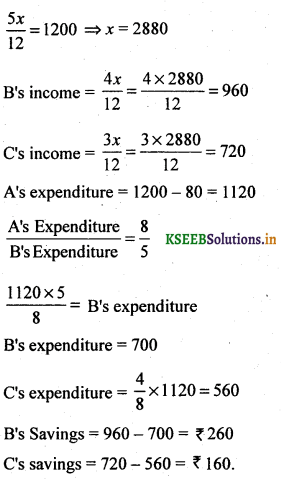# 2nd PUC Basic Maths Question Bank Chapter 7 Ratios and Proportions

Students can Download Basic Maths Question Bank Chapter 7 Ratios and Proportions Questions and Answers, Notes Pdf, 2nd PUC Basic Maths Question Bank with Answers helps you to revise the complete Karnataka State Board Syllabus and to clear all their doubts, score well in final exams.

## Karnataka 2nd PUC Basic Maths Question Bank Chapter 7 Ratios and Proportions

### 2nd PUC Basic Maths Ratios and Proportions One Mark Questions and Answers

Question 1.
If 3 : 5 is a ratio. Find the antecedent and consequent.
3 is antecedent and 5 is called consequent.

Question 2.
x gets a salary of Rs 20,000 y gets a salary of Rs. 5000. Find the ratio of their salaries.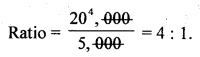Question 3.
Find the inverse ratio of 4 : 5.
5 : 4 is the Inverse ratio.Question 4.
Find the compound ratio of 3 : 4 and 4:7.
Compound ratio is
$$\frac{3}{4} \times \frac{4}{7}=\frac{3}{7}=3: 7$$

Question 5.
Find the duplicate ratio of 5 : 4.
52:42 = 25 : 16.

Question 6.
Find the triplicate ratio of 3 : 5.
33: 53 = 27 : 125.

Question 7.
Find the subduplicate ratio of 9 : 49.
$$\sqrt{9}: \sqrt{49}=3: 7$$Question 8.
Find the subtriplicate ratio of 125 : 64.
$$\sqrt{125}: \sqrt{64}=5: 4$$

Question 9.
Find the value of 5 : 20 = 3 :
5x = 20 x 3
$$x=\frac{20 \times 3}{5}=12$$

Question 10.
Raghu completes a job in 3 hrs and Mr Chakri complete the same job in 45 minutes, compare their performances.
3 hrs : 45 minutes                                                                                         –
3 x 60 minutes: 45 minutes
180 minutes: 45 minutes.Question 11.
Find the Fourth proportional to 4, 5,24.
Let x be the 4th proportion
4 : 5 : : 24 : x are in proportion
4x = 5 x 24
x = 30
∴ 4th proportion is 30.

Question 12.
Find the mean proportional to 2 and 8.
Let x be the mean proportion
∴ 2 : x : : x : 8
x2= 16 ⇒ x = 4.

Question 13.
Find the Third proportional to 3,12.
Let x be the third proportional
∴ 3 : 12 : : 12 : x are in proportion
3x = 144 ⇒ x = 48.Question 14.
Write the ratio of 8m to 3cm.
1 meter =100 cm, 8m = 800 cm
ratio is 800 : 3.

Question 15.
If a : b = 2 : 4 and b : c =5 then find a : c.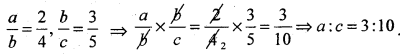Question 16.
Divide Rs. 7065 in the ratio 4 : 5
Sum of ratios is 9.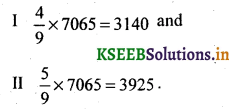Question 17.
If 3, x, 1083 are in continued proportion, Find
3 : x : : x : 1083
x2= 1083 x 3
x2 = 3249
⇒ x = 57.Question 18.
The Sum of 2 number is 1250, they are in the ratio 7 : 18. Find the numbers.
Let the 2 numbers be lx and 18x
Given 7x + 18x = 1250
25x = 1250 ⇒ x= 1250
The Two numbers are 7 x 50 = 350 and 18 x 50 = 400.

### 2nd PUC Basic Maths Ratios and Proportions Two Marks Questions and Answers

Question 19.
Two numbers whose difference is 176 are in the ratio 5 : 16. Find the numbers.
Let the 2 numbers be 5x and 16x
Given 16x – 5x= 176
11x = 176 ⇒ x = 16
∴ The two numbers is 5 x 16 and 16 x 16
80 and 256.

Question 20.
If 3a + b : 4a – 7b = 2 : 3 find a : b.
$$\frac{3 a+b}{4 a-7 b}=\frac{2}{3}$$
3(3a + b) = 2(4a – 7b)
9a + 3b = 8a – 14b
a = -17b
$$\frac{a}{b}=\frac{-17}{1}$$ ⇒ a : b = -17 : 1Question 21.
If a : b – 1 : 2 and b : c = 3 : 5 find a : b : c.
a : b = 1 : 2, b : c = 3 : 5
⇒ a : b : c = 3 : 6 : 10.

Question 22.
If 15 chairs cost Rs. 750. What will be the cost of 120 chairs at the same price.
Cost and chairs are in direct proportion
15 : 120 : : 750 : x
$$x=\frac{120 \times 750}{15}=6000$$
∴ Cost of 120 chairs are Rs. 6000.

Question 23.
Find the ratio between two numbers such that their sum is 40 and their difference is 8.
Let the two numbers be x and yQuestion 24.
Two numbers are in the ratio 3 : 5, If 5 is added to each, they are in the ratio 2 :3. Find the numbers?
Let the two numbers by 3x and 5x
Given $$\frac{3 x+5}{5 x+5}=\frac{2}{3}$$
9x + 15 = 10x+10
15 – 10= 10x – 9x ⇒ x = 5
∴ the two numbers are 5 and 5 x 5 = 15 & 25.Question 25.
What must be added to each term in the ratio 2 : 3 so that it becomes 5 : 6.
Let x must be added to 2 : 3
$$\frac{2+x}{3+x}=\frac{5}{6}$$
⇒ 15 + 5x =12 + 6x
15 – 12 = 6x – 5x
⇒ x = 3
∴ 3 must be added to each term in the ratio.

Question 26.
What must be substracted from each term in the ratio 7 : 4 so that it becomes 5 : 2. Sol. Answer:
Let x be substracted from each term of the ratio 7 : 4
∴ $$\frac{7-x}{4-x}=\frac{5}{2}$$
14 – 2x = 20 – 5x
5x – 2x = 20 – 14
3x = 6 ⇒  x = 2
∴ 2 must be substracted from each term in the ratio so that it becomes 5 : 2.

Question 27.
If a : b = 2 : 3, b : c = 3 : 5 and c : d= 5:7 find a : d ?Question 28.
If a : A = 2 : 3, b : c = 6 : 13 find a : b :c.
a : b = 2 : 3  ×  2
a : b = 4 : 6
b : c = 6 : 13
⇒ a : b : c = 4 : 6 : 13.Question 29.
H takes 3 hrs to pump out 2500 litres of water how much time will it take to pump out 15,000 litres?
Let the required time be ‘x’ hours
It is a case of direct proportions
3 : x = 2500 : 15000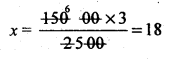∴ The time required to pump out 15,000 litres of water is 18 hours.

Question 30.
On a certain job one man worked 7 hours and another man worked 15 hours. If the job paid is Rs. 44. Divide the pay according to the number of hours each man had.
Total number of hrs worked = 7 hrs + 15 hrs = 22 hrs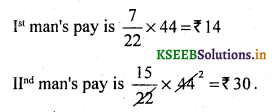### 2nd PUC Basic Maths Ratios and Proportions Three Marks Questions and Answers

Question 31.
Divide Rs. 1275 among A, B and C so that A: B = 3 : 4 and B : C = 2 : 5.
A : B : C = 6:8:20, Divide by 2
3 : 4 ⇒ 2 : 5
A : B : C = 3 : 4 : 10
Sum of the ratios =17
A’s share = $$\frac{3}{17}$$ x 1275 = 225
B’s share = $$\frac{4}{17}$$ x 1275 = 300
Cs share = $$\frac{10}{17}$$ x 1275 = 750.

Question 32.
Men, Women and boys are employed in a factory in the proportion of 3 : 1 : 2 and the wages of a man, a women and a boy are 4:3:2. When 45 men are employed the total daily wages of all amount to Rs. 1425. Find the daily wages paid to a man, women and a boy.
The ratio of men, women and boys = 3:1:2
Given the number of men = 45, women = 15, and no of boys = 30
Their wages are in the ratio 4 : 3 : 2
Let the wages of man = 4x, women = 15, and no of boys = 2x
Also given total daily wages = 1425
45(4x) + 15(3x) + 30 (2x) = 1425 ⇒ x = 5
The daily wage of a man = ₹ 20
The daily wage of a woman = ₹ 15
The daily wage of a boy =₹ 10.Question 33.
The ratio of the age of father to that of his son is 5 : 3. After 10 years, the ratio of their ages will be 3 : 2. Find their present ages.
Let father’s age be 5x*and sons age be 3x
After 10 years, father age = 10 + 5x, sons age = 10 + 3x
but the ratio of their ages is 3 : 2
$$\frac{10+5 x}{10+3 x}=\frac{3}{2}$$
20 + 10x = 30 + 9x ⇒ x = 10
The present father’s age is 5x = 5(10) = 50
The present father’s age is 3x = 3(10) = 30.

Question 34.
Divide ₹1800 in the ratio 3 : 4 : 5.
Let the 3 parts be x
3x + 4x + 5x = 1800
12x= 1800
∴ x= 150
1st part = 3x = 3 x 150= ₹450
2st part = 4x = 4 x 150 = ₹600
3rd part = 5x = 5 x 150 = ₹750.

Question 35.
If x : y = 3 : 4 find $$\frac{2 x^{2}+3 y^{2}}{x^{2}+y^{2}}$$Question 36.
If a : 6 = 2 : 3 and x : y = 4 : 7.Find $$\frac{5 a x+4 b y}{8 a x+3 b y}$$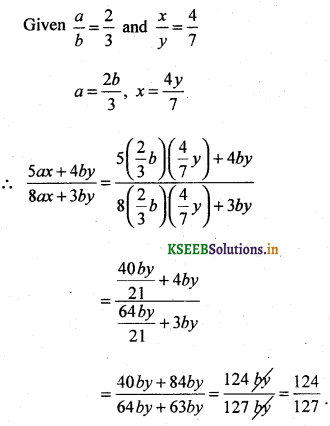Question 37.
The angles of a triangle are in the ratio 3:4:5. Find the angle’s.
Let the angles of the triangle be x
3x + 4x + 5x = 180
12x = 180
∴ x = 15°
The 3 angles are 3x = 45°, 4x = 60°, 5x = 15°.

Question 38.
An article is sold at 40% gain on the cost price. Find the ratio of the selling price and the cost price.
Let the cost price be x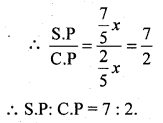Question 39.
If the monthly incomes of A and B are in the ratio 3 : 4 and their expenditures are in the ratio 1 : 2. If each saves ₹ 1000 find the monthly incomes.
Let the monthly incomes of A and B be 3x and 4x
we know that income – savings = expenditure
$$\frac{3 x-1000}{4 x-1000}=\frac{1}{2}$$
⇒ 6x – 2000 = 4x – 1 2x= 1000
⇒ x = 500
∴ The monthly incomes of A and B are ₹ 1500 and ₹ 2000.Question 40.
Two numbers are in the ratio 6 : 7 if the difference of their squares to 117. Find the numbers.
Let the two numbers be 6x and 7x
Given (7x)2 – (6x)2 =117
49x2 –  36x2 = 117
13x2 = 117
∴  x2 = 9 ⇒ x = ± 3
∴  The numbers are (3x and 6x) 18 and 21.

### 2nd PUC Basic Maths Ratios and Proportions Five Marks Questions and Answers

Question 41.
Divide ₹ 1800 into 3 parts, such that three times of the first, five times of the second and six times the third are equal.
Let the 3 parts be x, y, and z
Given 3x = 5y = 6z
3x = 5y ⇒ x : y = 5 : 3 …….. x 6
5y = 6z ⇒ y : z = 6:5 ………… x 3
x : y : z = 30 : 18 : 15 = 10 : 6 : 5
Sum of the ratios = 21
Ist part = $$\frac{10}{21}$$ x 1800 = 857.1428 ≈ 857
IInd part = $$\frac{6}{21}$$ x 1800 = 514 28 ≈ 514
IIIrd part = $$\frac{5}{21}$$ x 1800 = 428.57 ≈ 429

Question 42.
Divide ₹ 3262 among x,y, and Such that If ₹ 35, ₹ 15 and ₹ 12 are deducted from their respective shares, the remainders are in the ratio 3 : 5 : 8.
Let the shares be x, y, and z
Ratios of the remainders are 3 : 5 : 8
∴ x – 35 = 3a
y – 15 = 5a
z – 12 = 8a
⇒ x = 3a + 35
y = 5a + 15
z = 8a + 12
By the data x+y + z- 3262
⇒16a + 62 = 3262
⇒ $$a=\frac{3200}{16}=200$$
∴ the share’s of x = 35 + 3a = 35 + 600 = 635
y=15 + 5a=15 + 1000 = 1015
z = 12 + 8a = 12 + 1600 = 1612
∴Total of their shares = 3262.Question 43.
If x : y = 2 : 3 find the value of $$\frac{2 x^{3}+3 y^{3}}{x^{3}+y^{3}}$$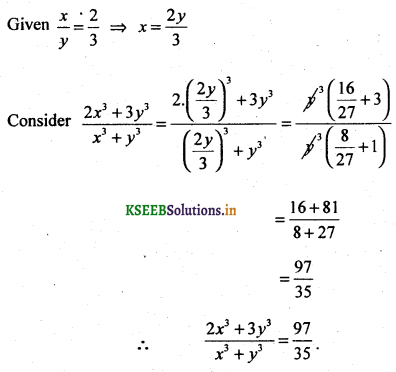Question 44.
Divide ₹ 5880 into 3 parts, such that B receive thrice as A & C receive of what $$\frac{5^{t h}}{6}$$
Let the 3 parts be x, y, and z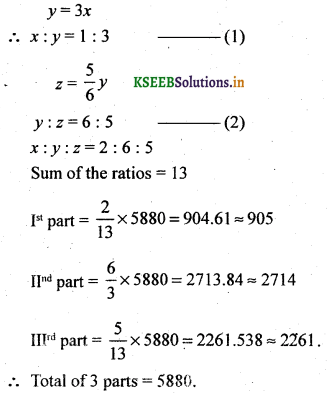Question 45.
Divide 6000 into 3 parts in the ratio $$\frac{1}{2}: \frac{1}{3}: \frac{1}{6}$$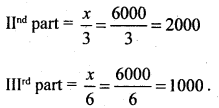Question 46.
The Raj dhani Express takes 18 hours to reach Delhi from Bhubaneshwar while Nilachala Express takes 24 hrs for the same of Delhi is 2880 kms from Bhubaneshwar find the ratio between the average speeds of the two trains.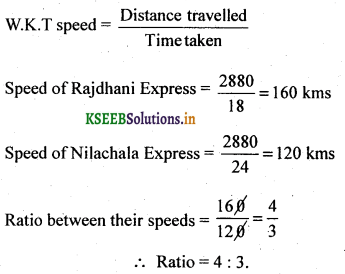Question 47.
Four numbers formed by adding 1, 5,10 & IS to a certain number are in proportion. Find the number.
Let the number be x
Four numbers formed be x + 1, x + 5, jc + 10 & x + 15 are in proportion
i.e. (x + 1), (x + 5), (x + 10): (x + 15)
$$\frac{x+1}{x+5}=\frac{x+10}{x+15}$$
x + 5 x + 15 (x+1)(x + 15) = (x + 5) (x +10) x2 + x + 15x + 15
= x2+5x+10x + 50
16x + 15 = 15x + 50
x = 35
∴ the four numbers are 35 + 1, 35 + 5, 35 + 10, 35 + 15 = 36, 40, 45, & 50.Question 48.
A can finish a job in 18 days and B can do the same job in 15 days. B worked for 10 days and left the job. In how many days, A alone can finish the remaining work?
A can finish the work in 18 days
A’s one day’s work = $$\frac{1}{18}$$
B’s one day’s work = $$\frac{1}{15}$$
B worked for days and left the job
B’s 10 days work = $$10 \times \frac{1}{15}=\frac{2}{3}$$
Remaining work = $$1-\frac{2}{3}=\frac{1}{3}$$
A alone requires =  $$\frac{1}{3}$$ x 18 = 6 days

Question 49.
Two laps can separately fill a tank in 12 and 15 minutes respectively. The tank when full can be emptied by a drain pipe in 20 minutes when the tank was empty, all the three were opened simultaneously. In what time will the tank be filled up.
Time taken to fill the tank is 12 and 15 minutes and time taken to drawn the tank is 20
minutes. In one minute the two taps will fill $$\frac{1}{12}, \frac{1}{15}$$ of the tank and drain $$\frac{1}{20}$$ of the tank.
∴ The required time will be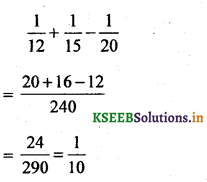Hence in one minute $$\frac{1}{10}$$ of the tank will be full the tank will be filled in minutes.

Question 50.
5 carpenters can earn Rs. 3600 in 6 days, working 9 hrs a day. How much will 8 carpenters earn in 12 days working 6 hrs a day.
It is a direct proportion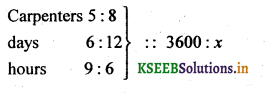8 carpenters earn Rs. 7680 in 12 days working 6 hrs a day.

Question 51.
16 men or 28 boys can fence a farm in 40 days. In how many days will 24 men and 14 boys. Complete the same work.
Given 16 mens work = 28 boy’s work
16 : 28
8 : 14
∴ 8 men = 14 boys
Now 24 men and 14 boys
= 24 men + 8 men
= 32 men
As the men increases, the days decreases it is an inverse proportion
1.6 : 32 = x : 40
32x = 16 x 40
x = 20
∴ 20 days are required to complete the work.Question 52.
2 men and 4 boys can do a work in 33 days, 3 men and 5 boys can do the same work in 24 days. How long shall 5 men and 2′ boys take to finish it?
2 men and 4 boys can work in 33 days
∴ In 1 any 66men and 132 boys can to the same work 3 men and 5 boys can do it in 24 days 72 men and 120 boys can do it in 1 day
∴ 66 men + 132 boys = 72 men + 120 boys 12 boys = 6 men
5 men & 2 boys are equivalent to 10 + 2 = 32 boys
8 : 12 = x : 33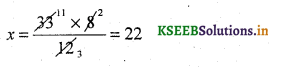5 men & 2 boys work in 22 days.

Question 53.
If 8 boys work in 33 days, 12 boys can work in less days. Monthly incomes of Raj u and Ravi are in the ratio 2 : 3. Their monthly expenditure are in the ratio 3 : 5. If each saves Rs. 1,000 per month. Find the monthly incomes of Raju and Ravi.
Let Raju income = 2x and Ravi’s income = 3x
Expenditure of Raju = 2x – 1000
Expenditure of Ravi = 3x -1000
By the data $$\frac{2 x-1000}{3 x-1000}=\frac{3}{5}$$
10x – 5000 = 9x -3000
x = 2000
Raja’s income = 2x = 2 x 2000 = 4000
Ravi’s income = 3x = 3 x 3000 = 6000.

Question 54.
The incomes of 3 persons A, B and C are in 5 : 4 : 3 and then spending are as 8 : 5 : 4. If A saves Rs 80 out of an income of Rs. 1200. Find the savings of B and C.